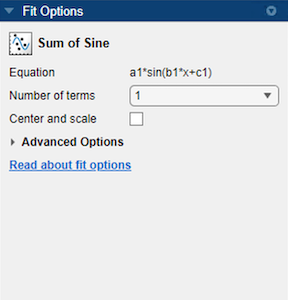Sum of Sines Models

The sum of sines model fits periodic functions, and is given by

$y=\sum _{i=1}^{n}{a}_{i}\mathrm{sin}\left({b}_{i}x+{c}_{i}\right)$

where a is the amplitude, b is the frequency, and c is the phase constant for each sine wave term. n is the number of terms in the series and 1 ≤ n ≤ 8. This equation is closely related to the Fourier series described in Fourier Series. The main difference is that the sum of sines equation includes the phase constant, and does not include a constant (intercept) term.

Fit Sum of Sine Models Interactively

1. Open the Curve Fitting app by entering cftool. Alternatively, click Curve Fitting on the Apps tab.

2. In the Curve Fitting app, select curve data (X data and Y data, or just Y data against index).

Curve Fitting app creates the default curve fit, Polynomial.

3. Change the model type from Polynomial to Sum of Sine.You can specify the following options:

• Choose the number of terms: 1 to 8.

Look in the Results pane to see the model terms, the values of the coefficients, and the goodness-of-fit statistics.

• (Optional) Click Fit Options to specify coefficient starting values and constraint bounds, or change algorithm settings.

The toolbox calculates optimized start points for sum of sine models, based on the current data set. You can override the start points and specify your own values in the Fit Options dialog box.

The sum of sine model has a lower bound constraint on ci of 0. The default lower bounds for most library models are -Inf.

For more information on the settings, see Specifying Fit Options and Optimized Starting Points.

Selecting a Sum of Sine Fit at the Command Line

Specify the model type sin followed by the number of terms, e.g., 'sin1' to 'sin8'.

For example, to load some periodic data and fit a six-term sum of sine model:

f = fit( month, pressure, 'sin6')
plot(f,month,pressure)

If you want to modify fit options such as coefficient starting values and constraint bounds appropriate for your data, or change algorithm settings, see the table of additional properties with NonlinearLeastSquares on the fitoptions reference page.